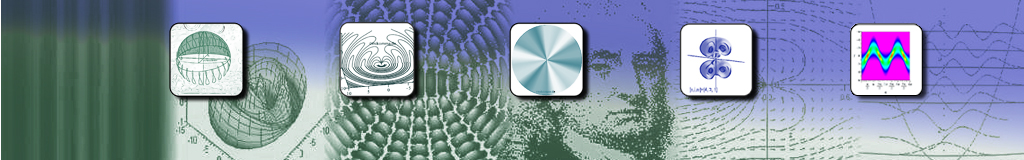Feynmans Herleitung der Schrödingergleichung

Text auszugsweise aus

Feynman and Hibbs Quantum Mechanics and Path Integrals,
McGraw-Hill Publishing Company 1965.  4. Kapitel

Formeln (blau): Die "Rechnung" wurde mit Maple symbolisch durchgeführt.

4 The Schrödinger Description of Quantum Mechanics

The path integrals which we have discussed so far have integrands which are exponentials of actions with the property (4-1)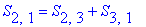Such path integrals can be analyzed in terms of the properties of integral equations which can be deduced from them. [...]

A still more convenient method is to reduce the path integrals to differential equations if possible. This possibility exists in quantum mechanics and is, in fact, the most convenient way to present that theory. It is in almost every case easier to solve the differential equation than it is to evaluate the path integral. The conventional presentation of quantum mechanics is based on this equation, called the Schrödinger equation. Here we shall derive this equation from our formulation. [...]

In this chapter our purpose is twofold: (1) For the reader primarily interested in quantum mechanics our aim is to connect the path integral formulation with other formulations which are found in the standard literature [...]. (2) For the reader primarily interested in path integrals this chapter will show a technique which is available for a certain class of path integrals to reduce these path integrals to differential equations. This technique is best shown by the particular example of quantum mechanics which we shall develop here.

THE SCHRÖDINGER EQUATION

The Differential Equation Form. The reason that we can develop a differential equation is that the relationship of Eq. (4-1) is correct for any values of the points 1, 2 and 3. For example, the time can be only an infinitesimal timegreater than the time. This will permit us to relate the value of a path integral for one time to its value a short time later. In this manner we can obtain a differential equation for the path integral.

We have already found that as a consequence of Eq. (4-1) we can define a wave function. Furthermore, we know that the equation (4-2)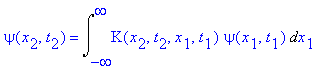gives the wave function at a timein terms of the wave function at a time. In order to obtain the differential equation we seek, we apply this relationship in the special case that the time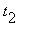differs only by an infinitesimal interval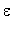from. The kernel K(2,1) is proportional to the exponential of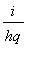(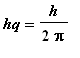) times the action for the interval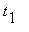to. For a short intervalthe action is approximatelytimes the lagragian for this interval. That is, using the same approximation as that of Eq. (2-34)we have (4-3)We shall now apply this  to the special case of a particle moving in a potential V(x,t) in one dimension, i.e., that for whichIn this case Eq. (4-3) becomes (4-4)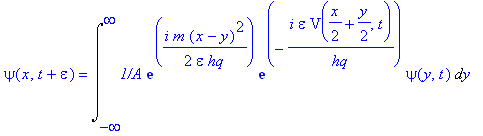The quantity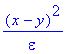appears in the exponent of the first factor. It is clear that if y is appreciably differentfrom x, this quantity is very large and the exponential consequently oscillates very rapidly as y varies. When this factor oscillates rapidly, the integral over y gives a very small value (because of the smooth behavior of the other factors). Only if y is near x (where the exponential changes more slowly) do we get important contributions. For this reason we make the substitution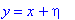with the expectation that appreciable contributions to the integral will occur only for small. We obtain  (4-5)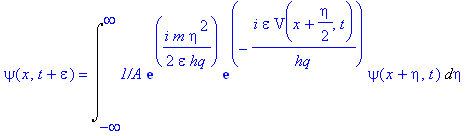The phase of the first exponential changes by the order of 1 radian when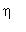is of the order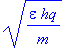so that most of the integral is contributed by values ofin this order.

We may expand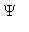in a power series. We need only keep terms of order. This implies keeping second-order terms in. The termmay be replaced by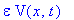because the error is of higher order than. Expanding the left-hand side to first order inand the right-hand side to  first order inand second order in, we obtain (4-6)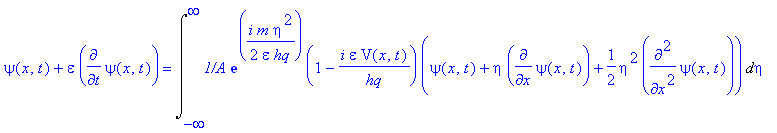If we take the leading term on the right-hand side, we have the quantity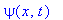multiplied by the integral (4-7)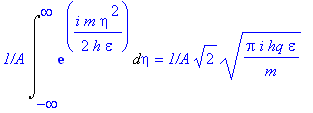On the left-hand side we just have. In order  that both sides agree in the limitapproaches 0, it is necessary that A  be so chosen that the expression of Eq. (4-7) equals 1. That is (4-8),[...]. This is a way of obtaining the quantity A in more complicated problems also. The A must be so chosen that the equation is correct to zero order in. Otherwise, no limit will exist asapproaches 0 in the original path integral.

In order to evaluate the right-hand side of Eq. (4-6), we shall have to use two integrals  (4-9)and (4-10)Writing out the right-hand side of Eq. (4-6) gives (4-11)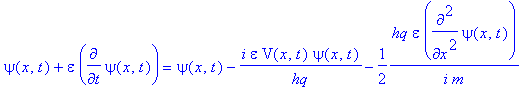This will be true to orderif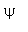satisfies the differential equation (4-12)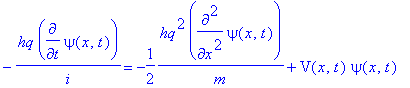This is the Schrödinger equation for our problem of a particle moving in one dimension. Corresponding equations in more complicated situations can be worked out in the same way.